Electron. J. Diff. Eqns., Vol. 2008(2008), No. 161, pp. 1-7.

### Asymptotic expansion formulas for the maximum of solutions to diffusive logistic equations Tetsutaro Shibata

Abstract:
We consider the nonlinear eigenvalue problems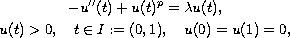where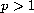is a constant and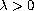is a parameter. This equation is well known as the original logistic equation of population dynamics when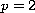. We establish the precise asymptotic formula for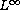-norm of the solution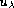as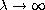whenand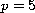.

Submitted May 07, 2008. Published December 9, 2008.
Math Subject Classifications: 34B15.
Key Words: Logistic equation;-norm of solutions.

Show me the PDF file (197 KB), TEX file, and other files for this article.Tetsutaro Shibata Department of Applied Mathematics Graduate School of Engineering, Hiroshima University Higashi-Hiroshima, 739-8527, Japan email: shibata@amath.hiroshima-u.ac.jp